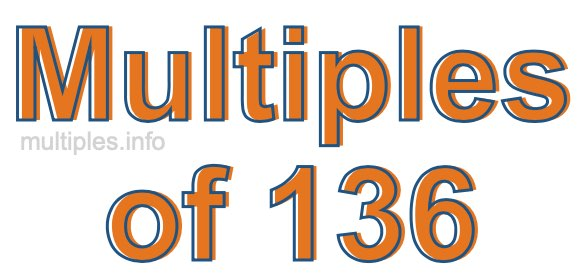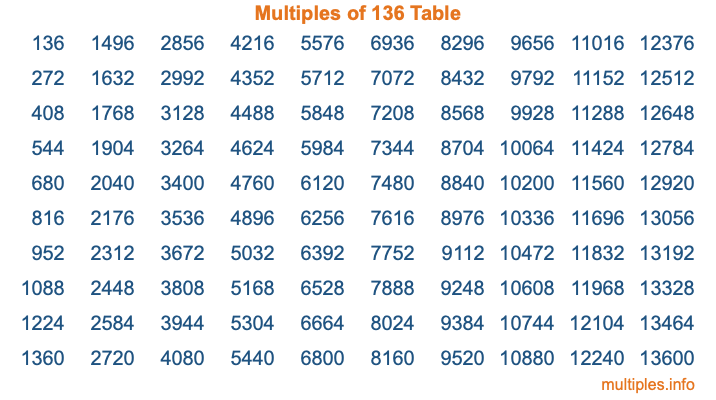Multiples of 136Welcome to the Multiples of 136 page. Here we will first teach you everything you will ever need to know about the multiples of 136, and then give you a study guide summary of everything we taught you to make sure you remember it all. Use this page to look up facts and learn information about the multiples of 136. This page will make you a multiples of one hundred thirty-six expert!

Definition of Multiples of 136
Multiples of 136 are all the numbers that when divided by 136 equal an integer. Each of the multiples of 136 are called a multiple. A multiple of 136 is created by multiplying 136 by an integer.

Therefore, to create a list of multiples of 136, you start with 1 multiplied by 136, then 2 multiplied by 136, then 3 multiplied by 136, and so on for as long as you want. Thus, the list of the first five multiples of 136 is 136, 272, 408, 544, and 680. To see a larger list of multiples of 136, see the printable image of Multiples of 136 further down on this page. We also have a category where you can choose any nth multiple of 136.

Multiples of 136 Checker
The Multiples of 136 Checker below checks to see if any number of your choice is a multiple of 136. In other words, it checks to see if there is any number (integer) that when multiplied by 136 will equal your number. To do that, we divide your number by 136. If the the quotient is an integer, then your number is a multiple of 136.

Is  a multiple of 136?

Least Common Multiple of 136 and ...
A Least Common Multiple (LCM) is the lowest multiple that two or more numbers have in common. This is also called the smallest common multiple or lowest common multiple and is useful to know when you are adding our subtracting fractions. Enter one or more numbers below (136 is already entered) to find the LCM.

Check out our LCM Calculator if you need more details about the Least Common Multiple or if you need the LCM for different numbers for adding and subtraction fractions.

nth Multiple of 136
As we stated above, 136 is the first multiple of 136, 272 is the second multiple of 136, 408 is the third multiple of 136, and so on. Enter a number below to find the nth multiple of 136.

th multiple of 136

Multiples of 136 vs Factors of 136
136 is a multiple of 136 and a factor of 136, but that is where the similarities end. All postive multiples of 136 are 136 or greater than 136. All positive factors of 136 are 136 or less than 136.

Below is the beginning list of multiples of 136 and the factors of 136 so you can compare:

Multiples of 136: 136, 272, 408, 544, 680, etc.

Factors of 136: 1, 2, 4, 8, 17, 34, 68, 136

As you can see, the multiples of 136 are all the numbers that you can divide by 136 to get a whole number. The factors of 136, on the other hand, are all the whole numbers that you can multiply by another whole number to get 136.

It's also interesting to note that if a number (x) is a factor of 136, then 136 will also be a multiple of that number (x).

Multiples of 136 vs Divisors of 136
The divisors of 136 are all the integers that 136 can be divided by evenly. Below is a list of the divisors of 136.

Divisors of 136: 1, 2, 4, 8, 17, 34, 68, 136

The interesting thing to note here is that if you take any multiple of 136 and divide it by a divisor of 136, you will see that the quotient is an integer.

Multiples of 136 Table
Below is an image of the first 100 multiples of 136 in a table. The table is in chronological order, column by column. The first column has the first ten multiples of 136, the second column has the next ten multiples of 136, and so on.The Multiples of 136 Table is also referred to as the 136 Times Table or Times Table of 136. You are welcome to print out our table for your studies.

Negative Multiples of 136
Although not often discussed or needed in math, it is worth mentioning that you can make a list of negative multiples of 136 by multiplying 136 by -1, then by -2, then by -3, and so on, to get the following list of negative multiples of 136:

-136, -272, -408, -544, -680, etc.

Multiples of 136 Summary
Below is a summary of important Multiples of 136 facts that we have discussed on this page. To retain the knowledge on this page, we recommend that you read through the summary and explain to yourself or a study partner why they hold true.

There are an infinite number of multiples of 136.

A multiple of 136 divided by 136 will equal a whole number.

136 divided by a factor of 136 equals a divisor of 136.

The nth multiple of 136 is n times 136.

The largest factor of 136 is equal to the first positive multiple of 136.

136 is a multiple of every factor of 136.

136 is a multiple of 136.

A multiple of 136 divided by a divisor of 136 equals an integer.

136 divided by a divisor of 136 equals a factor of 136.

Any integer times 136 will equal a multiple of 136.

Multiples of a Number
Here you can get the multiples of another number, all with the same attention to detail as we did for multiples of 136 on this page.

Multiples of
Multiples of 137
Did you find our page about multiples of one hundred thirty-six educational? Do you want more knowledge? Check out the multiples of the next number on our list!